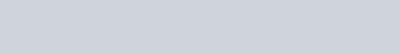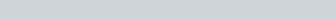Finding the equation of the curve given dy/dx

In this video, I show you how to find the equation of a curve and the constant of integration through this example.

Example:

1.Finding the equation of a curve from its gradient function | Tutorial 1 | ExamSolutions - youtube Video

Finding the equation of the curve given f'(x)

In this video, I show you how to find f(x) and the constant of integration through another example.

Example:

1.Finding the equation of a curve from its gradient function | Tutorial 2 | ExamSolutions - youtube Video